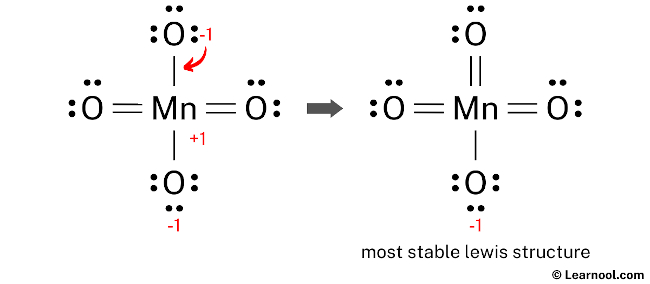# MnO4- Lewis structure

MnO4 (permanganate) has one manganese atom and four oxygen atoms.

In the MnO4 Lewis structure, there is one single bond and three double bonds around the manganese atom, with four oxygen atoms attached to it. The oxygen atom with a single bond has three lone pairs, and the oxygen atom with double bonds has two lone pairs.

Also, there is a negative (-1) charge on the oxygen atom with a single bond.

Contents

## Steps

Here’s how you can easily draw the MnO4 Lewis structure step by step:

#1 Draw a rough skeleton structure
#2 Mention lone pairs on the atoms
#3 If needed, mention formal charges on the atoms
#4 Minimize formal charges by converting lone pairs of the atoms, and try to get a stable Lewis structure
#5 Repeat step 4 again if needed, until all charges are minimized

Now, let’s take a closer look at each step mentioned above.

### #1 Draw a rough skeleton structure

• First, determine the total number of valence electrons

In the periodic table, manganese is a transition metal having an electron configuration [Ar] 3d54s2, so manganese has seven valence electrons. And oxygen lies in group 16, so oxygen has six valence electrons.

Since MnO4 has one manganese atom and four oxygen atoms, so…

Valence electrons of one manganese atom = 7 × 1 = 7
Valence electrons of four oxygen atoms = 6 × 4 = 24

Now the MnO4 has a negative (-1) charge, so we have to add one more electron.

So the total valence electrons = 7 + 24 + 1 = 32

• Second, find the total electron pairs

We have a total of 32 valence electrons. And when we divide this value by two, we get the value of total electron pairs.

Total electron pairs = total valence electrons ÷ 2

So the total electron pairs = 32 ÷ 2 = 16

• Third, determine the central atom

We have to place the least electronegative atom at the center.

Since manganese is less electronegative than oxygen, assume that the central atom is manganese.

Therefore, place manganese in the center and oxygens on either side.

• And finally, draw the rough sketch

### #2 Mention lone pairs on the atoms

Here, we have a total of 16 electron pairs. And four Mn — O bonds are already marked. So we have to only mark the remaining twelve electron pairs as lone pairs on the sketch.

Also remember that manganese is a period 4 element, so it can keep more than 8 electrons in its last shell. And oxygen is a period 2 element, so it can not keep more than 8 electrons in its last shell.

Always start to mark the lone pairs from outside atoms. Here, the outside atoms are oxygens.

So for each oxygen, there are three lone pairs, and for manganese, there is zero lone pair because all twelve electron pairs are over.

Mark the lone pairs on the sketch as follows:

### #3 If needed, mention formal charges on the atoms

Use the following formula to calculate the formal charges on atoms:

Formal charge = valence electrons – nonbonding electrons – ½ bonding electrons

For manganese atom, formal charge = 7 – 0 – ½ (8) = +3

For each oxygen atom, formal charge = 6 – 6 – ½ (2) = -1

Here, both manganese and oxygen atoms have charges, so mark them on the sketch as follows:

The above structure is not a stable Lewis structure because both manganese and oxygen atoms have charges. Therefore, reduce the charges (as below) by converting lone pairs to bonds.

### #4 Minimize formal charges by converting lone pairs of the atoms

Convert a lone pair of the oxygen atom to make a new Mn — O bond with the manganese atom as follows:

### #5 Since there are charges on atoms, repeat step 4 again

Since there are charges on manganese and oxygen atoms, again convert a lone pair of the oxygen atom to make a new Mn — O bond with the manganese atom as follows:

### #6 Minimize charges again

There are still charges on manganese and oxygen atoms, so again convert a lone pair of the oxygen atom to make a new Mn — O bond with the manganese atom as follows:Lone pair of top oxygen is converted, and got the most stable Lewis structure of MnO4–

In the above structure, you can see that the central atom (manganese) forms an octet. And the outside atoms (oxygens) also form an octet. Hence, the octet rule is satisfied.

Now there is still a negative (-1) charge on the oxygen atom.

This is okay, because the structure with a negative charge on the most electronegative atom is the best Lewis structure. And in this case, the most electronegative element is oxygen.

Also, the above structure is more stable than the previous structures. Therefore, this structure is the most stable Lewis structure of MnO4.

And since the MnO4 has a negative (-1) charge, mention that charge on the Lewis structure by drawing brackets as follows: